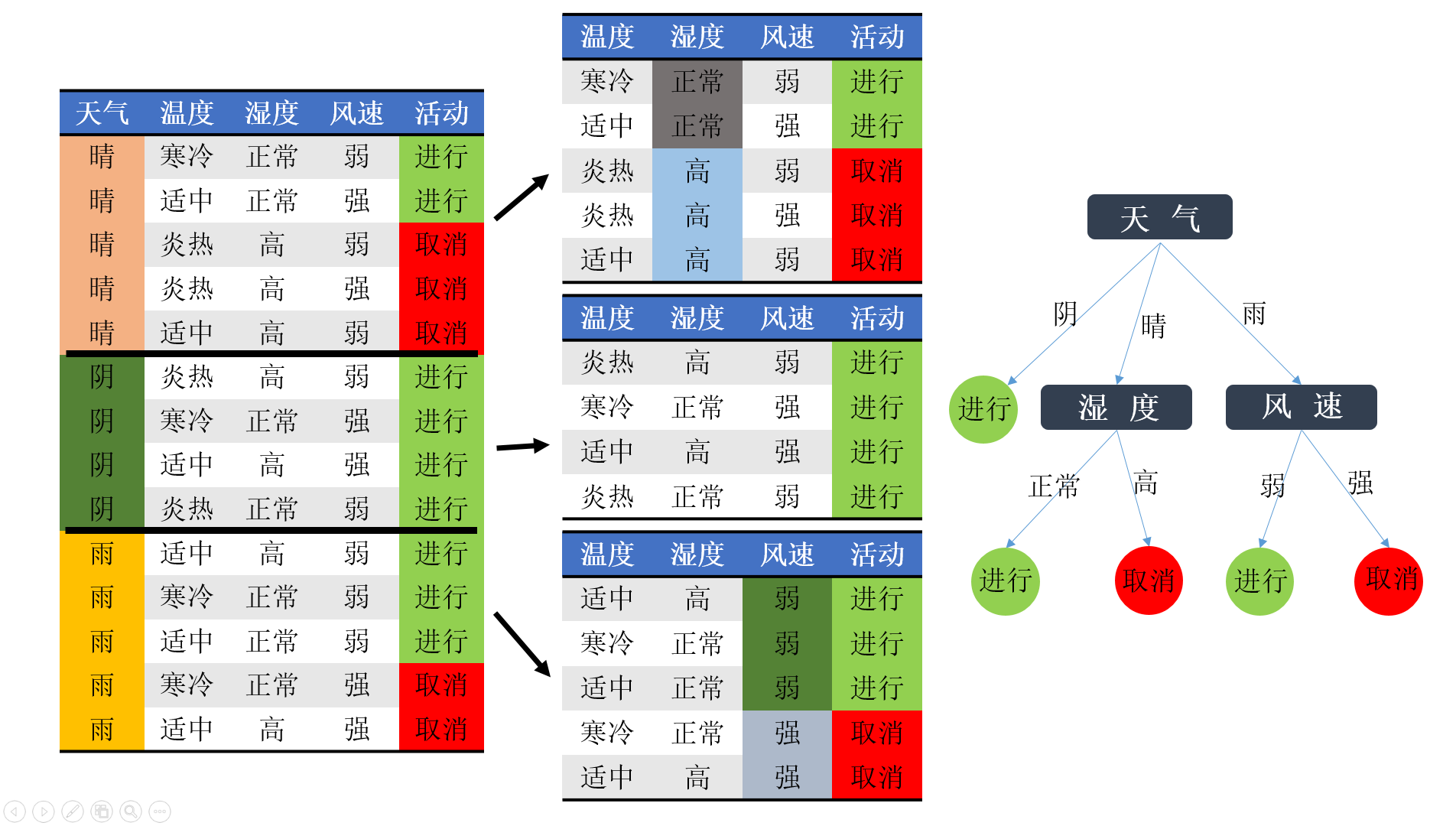决策树：ID3算法与实现

信道模型和信息的含义

1. 通信过程是在随机干扰的环境汇中传递信息的过程
2. 信宿对于信源的先验不确定性：在通信前，信宿不能确切的了解信源的状态；
3. 信宿对于信源的后验不确定性：在通信后，由于存在干扰，信宿对于接收到的信息仍然具有不确定性
4. 后验不确定性总是要小于先验不确定性的。

信息的定量描述

1. f(p)应该是概率p的严格单调递减函数，
2. 当p=1时，f(p)=0
3. 当p=0时，f(p)=
4. 两个独立事件的联合信息量应该等于它们信息量之和。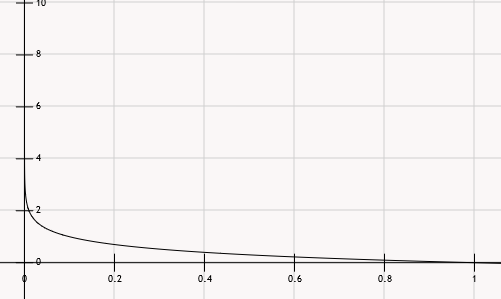自信息和熵的定义

I=log(p)

1. 当该消息发生之前，表示发生该消息的不确定性，
2. 当该消息发生之后，表示消息所含有的信息量。

H(X)=iNp(xi)I(xi)=iNp(xi)logp(xi)

I(xi|yj)=logp(xi|yj)

H(X|yj)=iNp(xi|yj)I(xi|yj)=iNp(xi|yj)log(p(xi|yj))

H(X|Y)=jp(yj)H(X|yj)=jp(yj)ip(xi|yj)logp(xi|yj)

I(X,Y)=H(X)H(X|Y)

例子

H()=914log914514log514=0.94

(总的步骤是计算先验熵，在计算后验熵，在计算条件熵。现在先验熵已知)

H(|)=25log2535log35=0.971

H(|)=1log10log0=0

H(|)=35log3525log25=0.971

H(|)=514H(|)+414H(|)+514H(|)=0.693

I()=H()H(|)=0.246

1. ID3算法

1. 计算先验熵，没有接收到其他的属性值时的平均不确定性，
2. 计算后验墒，在接收到输出符号yi时关于信源的不确定性，
3. 条件熵，对后验熵在输出符号集Y中求期望，接收到全部的付好后对信源的不确定性，
4. 互信息，先验熵和条件熵的差，

实例

1.计算先验熵：在没有接收到其他的任何的属性值时候，活动进行与否的熵根据下表进行计算。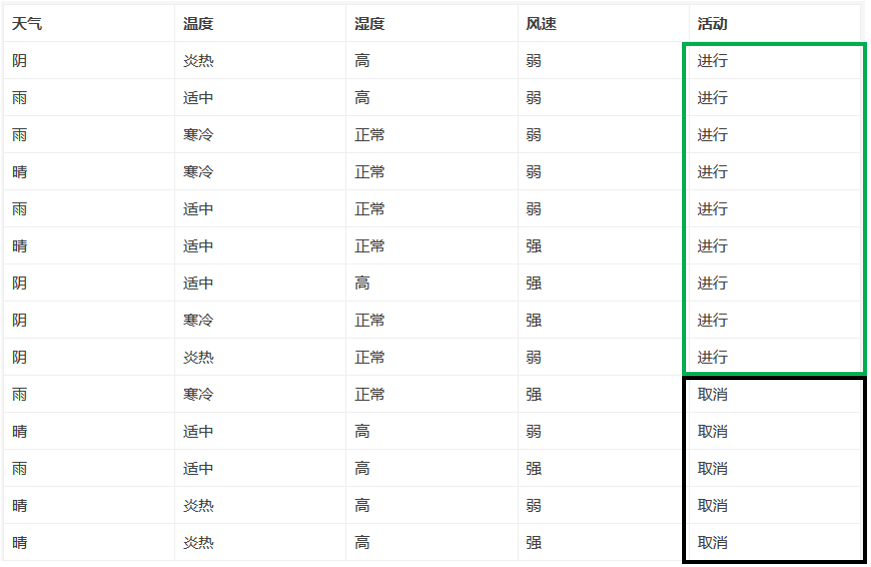H()=914log914514log514=0.94

2.分别将各个属性作为决策属性时的条件熵（先计算后验墒，在计算条件熵）

(1) 计算已知天气情况下活动是否进行的条件熵（已知天气情况下对于活动的不确定性）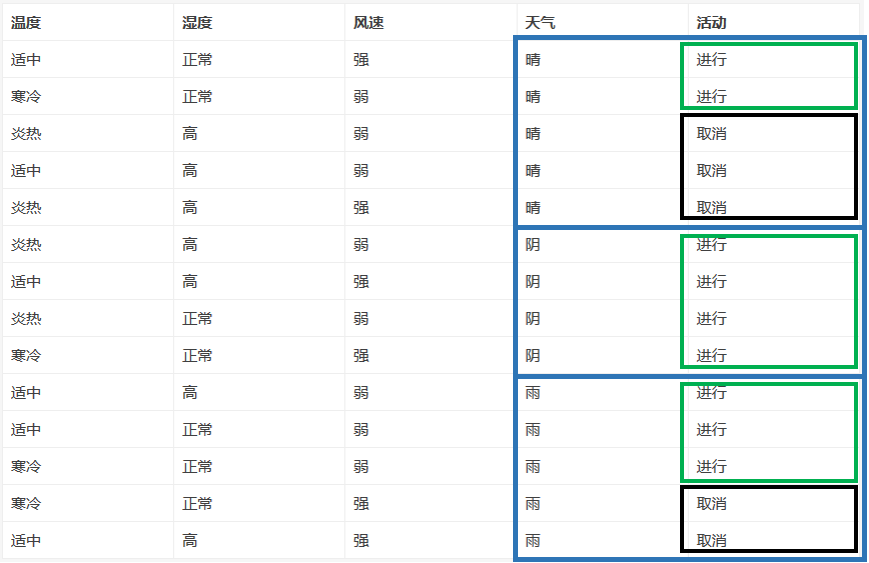H(|)=P(|)logP(|)P(|)logP(|)=0.971

H(|)=P(|)logP(|)P(|)logP(|)=0

H(|)=P(|)logP(|)P(|)logP(|)=0.971

H|=5/14H|+4/14H|+5/14H|=0.693

(2)计算已知温度情况时对活动的条件熵(不确定性)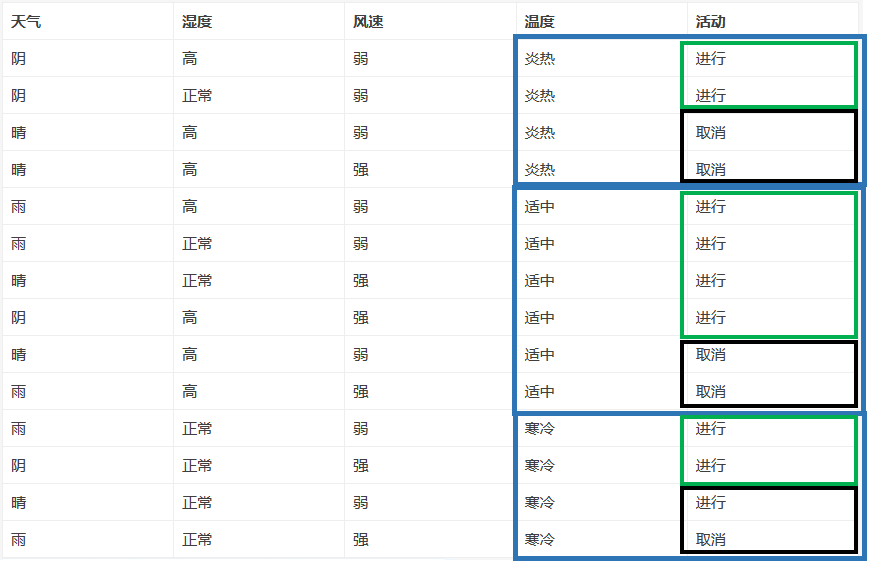H(|)=0.911

(3)已知湿度情况下对于活动是否进行的条件熵（不确定性）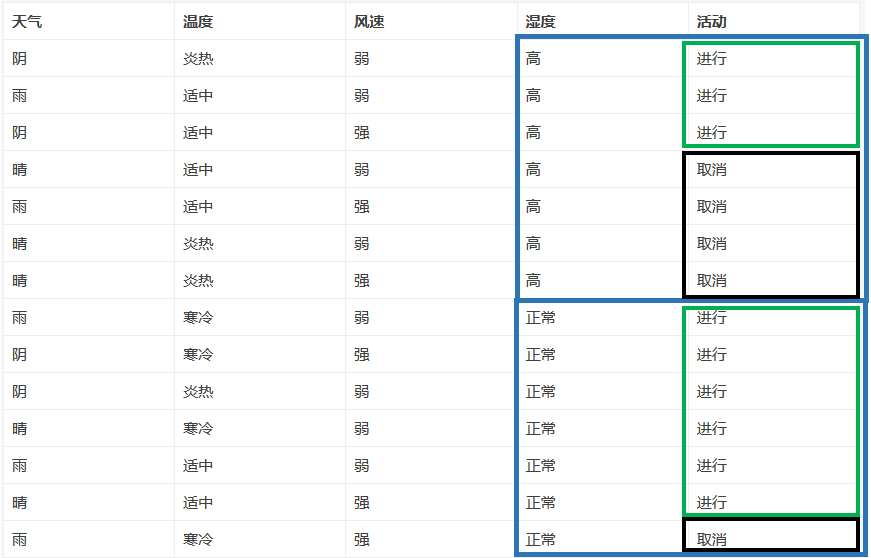H(|湿)=0.789

(4)已知风速情况下对于活动是否进行的条件熵（不确定性）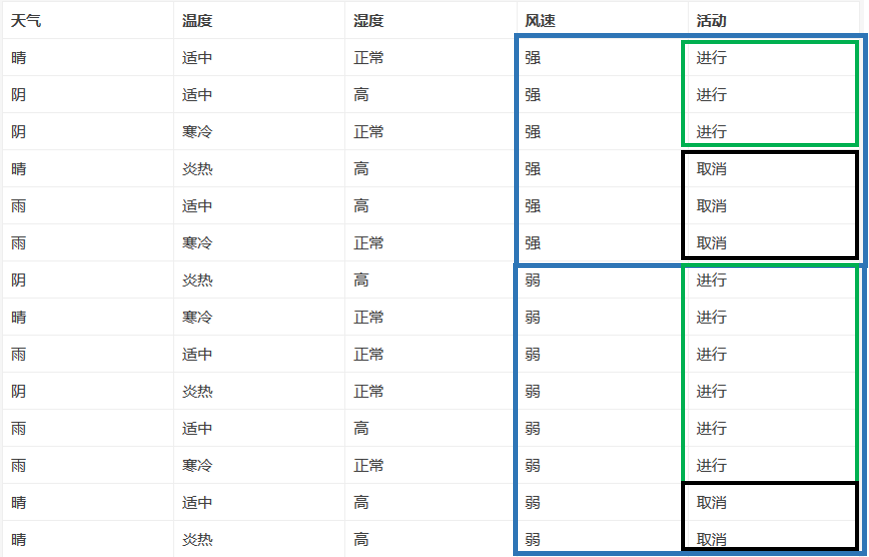H(|)=0.892

3.计算信息增益
I()=H()H(|)=0.940.693=0.246

I()=H()H(|)=0.940.911=0.029

I(湿)=H()H(|湿)=0.940.789=0.151

I()=H()H(|)=0.940.892=0.048